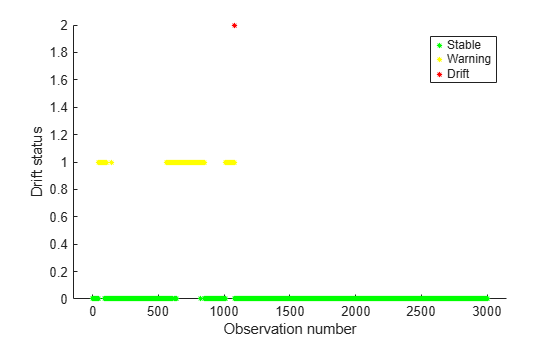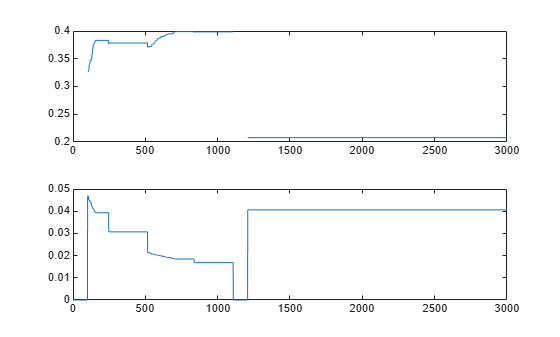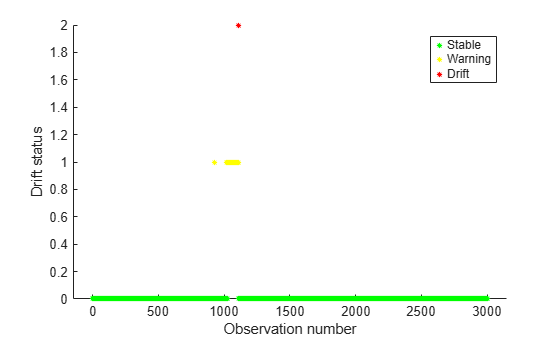# incrementalConceptDriftDetector

Instantiate incremental concept drift detector

## Syntax

``IncCDDetector = incrementalConceptDriftDetector()``
``IncCDDetector = incrementalConceptDriftDetector(DetectionMethod)``
``IncCDDetector = incrementalConceptDriftDetector(DetectionMethod,Name=Value)``

## Description

````IncCDDetector = incrementalConceptDriftDetector()` returns an incremental concept drift detector that utilizes the default method, Hoeffding's Bounds Drift Detection Method with moving average test (HDDMA).```

example

````IncCDDetector = incrementalConceptDriftDetector(DetectionMethod)` returns an incremental concept drift detector that utilizes the method `DetectionMethod`.```

example

````IncCDDetector = incrementalConceptDriftDetector(DetectionMethod,Name=Value)` specifies additional options using one or more `Name=Value` arguments.```

## Examples

collapse all

Initiate the concept drift detector using the Drift Detection Method (DDM).

`incCDDetector = incrementalConceptDriftDetector("ddm");`

Create a random stream such that for the first 1000 observations, failure rate is 0.1 and after 1000 observations, failure rate increases to 0.6.

```rng(1234) % For reproducibility numObservations = 3000; switchPeriod = 1000; for i = 1:numObservations if i <= switchPeriod failurerate = 0.1; else failurerate = 0.6; end X(i) = rand()<failurerate; % Value 1 represents failure end```

Preallocate variables for tracking drift status.

```status = zeros(numObservations,1); statusname = strings(numObservations,1);```

Continuously feed the data to the drift detector and perform incremental drift detection. At each iteration:

• Update statistics of the drift detector and monitor for drift using the new data point with `detectdrift`. (Note: `detectdrift` checks for drift after the warmup period.)

• Track and record the drift status for visualization purposes.

• When a drift is detected, reset the incremental concept drift detector by using `reset`.

```for i = 1:numObservations incCDDetector = detectdrift(incCDDetector,X(i)); statusname(i) = string(incCDDetector.DriftStatus); if incCDDetector.DriftDetected status(i) = 2; incCDDetector = reset(incCDDetector); % If drift detected, reset the detector sprintf("Drift detected at Observation #%d. Detector reset.",i) elseif incCDDetector.WarningDetected status(i) = 1; else status(i) = 0; end end```
```ans = "Drift detected at Observation #1078. Detector reset." ```

After the change in the failure rate at observation number 1000, `detectdrift` detects the shift at observation number 1078.

Plot the drift status versus the observation number.

`gscatter(1:numObservations,status,statusname,'gyr','*',4,'on',"Observation number","Drift status")`Create a random stream such that the observations come from a normal distribution with standard deviation 0.75, but the mean changes over time. First 1000 observations come from a distribution with mean 2, the next 1000 come from a distribution with mean 4, and the following 1000 come from a distribution with mean 7.

```rng(1234) % For reproducibility numObservations = 3000; switchPeriod1 = 1000; switchPeriod2 = 2000; X = zeros([numObservations 1]); % Generate the data for i = 1:numObservations if i <= switchPeriod1 X(i) = normrnd(2,0.75); elseif i <= switchPeriod2 X(i) = normrnd(4,0.75); else X(i) = normrnd(7,0.75); end end```

In an incremental drift detection application, access to data stream and model update would happen consecutively. One would not collect the data first and then feed into the model. However, for the purpose of clarification, this example demonstrates the simulation of data separately.

Specify the drift warmup period as 50 observations and estimation period for the data input bounds as 100.

```driftWarmupPeriod = 50; estimationPeriod = 100;```

Initiate the incremental concept drift detector. Utilize the Hoeffding's bounds method with exponentially weighted moving average method (EWMA). Specify the input type and warmup period.

```incCDDetector = incrementalConceptDriftDetector("hddmw",InputType="continuous", ... WarmupPeriod=driftWarmupPeriod,EstimationPeriod=estimationPeriod)```
```incCDDetector = HoeffdingDriftDetectionMethod PreviousDriftStatus: 'Stable' DriftStatus: 'Stable' IsWarm: 0 NumTrainingObservations: 0 Alternative: 'greater' InputType: 'continuous' TestMethod: 'ewma' Properties, Methods ```

`incDDetector` is a `HoeffdingDriftDetectionMethod` object. When you first create the object, properties such as `DriftStatus`, `IsWarm`, `CutMean`, and `NumTrainingObservations` are at their initial state. `detectdrift` updates them as you feed the data incrementally and monitor for drift.

Preallocate the batch size and the variables to record drift status and the mean the drift detector computes with each income of data.

```status = zeros([numObservations 1]); statusname = strings([numObservations 1]); M = zeros([numObservations 1]);```

Simulate the data stream of one observation at a time and perform incremental drift detection. At each iteration:

• Monitor for drift using the new data with `detectdrift`.

• Track and record the drift status and the statistics for visualization purposes.

• When a drift is detected, reset the incremental concept drift detector by using the function `reset`.

```for i = 1:numObservations incCDDetector = detectdrift(incCDDetector,X(i)); M(i) = incCDDetector.Mean; if incCDDetector.DriftDetected status(i) = 2; statusname(i) = string(incCDDetector.DriftStatus); incCDDetector = reset(incCDDetector); % If drift detected, reset the detector sprintf("Drift detected at observation #%d. Detector reset.",i) elseif incCDDetector.WarningDetected status(i) = 1; statusname(i) = string(incCDDetector.DriftStatus); sprintf("Warning detected at observation #%d.",i) else status(i) = 0; statusname(i) = string(incCDDetector.DriftStatus); end end```
```ans = "Warning detected at observation #1024." ```
```ans = "Warning detected at observation #1025." ```
```ans = "Warning detected at observation #1026." ```
```ans = "Warning detected at observation #1027." ```
```ans = "Warning detected at observation #1028." ```
```ans = "Warning detected at observation #1029." ```
```ans = "Drift detected at observation #1030. Detector reset." ```
```ans = "Warning detected at observation #2012." ```
```ans = "Warning detected at observation #2013." ```
```ans = "Warning detected at observation #2014." ```
```ans = "Drift detected at observation #2015. Detector reset." ```

Plot the drift status versus the observation number.

`gscatter(1:numObservations,status,statusname,'gyr','*',5,'on',"Number of observations","Drift status")`Plot the mean values versus the number of observations.

`scatter(1:numObservations,M)`You can see the increase in the sample mean from the plot. The mean value becomes larger and the software eventually detects the drift in the data. Once a drift is detected, reset the incremental drift detector. This also resets the mean value. In the plot, the observations where the sample mean is zero correspond to the estimation periods. There is an estimation period at the beginning and then twice after the drift detector is reset following the detection of a drift.

Initiate the concept drift detector using the Drift Detection Method (DDM).

`incCDDetector = incrementalConceptDriftDetector("ddm",Alternative="less",WarmupPeriod=100);`

Create a random stream such that for the first 1000 observations, failure rate is 0.4 and after 1000 failure rate decreases to 0.1.

```rng(1234) % For reproducibility numObservations = 3000; switchPeriod = 1000; for i = 1:numObservations if i <= switchPeriod failurerate = 0.4; else failurerate = 0.125; end X(i) = rand()<failurerate; % Value 1 represents failure end```

Preallocate variables for tracking drift status and the optimal mean and optimal standard deviation value.

```optmean = zeros(numObservations,1); optstddev = zeros(numObservations,1); status = zeros(numObservations,1); statusname = strings(numObservations,1);```

Continuously feed the data to the drift detector and monitor for any potential change. Record the drift status for visualization purposes.

```for i = 1:numObservations incCDDetector = detectdrift(incCDDetector,X(i)); statusname(i) = string(incCDDetector.DriftStatus); optmean(i) = incCDDetector.OptimalMean; optstddev(i) = incCDDetector.OptimalStandardDeviation; if incCDDetector.DriftDetected status(i) = 2; incCDDetector = reset(incCDDetector); % If drift detected, reset the detector sprintf("Drift detected at Observation #%d. Detector reset.",i) elseif incCDDetector.WarningDetected status(i) = 1; else status(i) = 0; end end```
```ans = "Drift detected at Observation #1107. Detector reset." ```

After the change in the failure rate at observation number 1000, `detectdrift` detects the shift at observation number 1096.

Plot the change in the optimal mean and optimal standard deviation.

```tiledlayout(2,1); ax1 = nexttile; plot(ax1,1:numObservations,optmean) ax2 = nexttile; plot(ax2,1:numObservations,optstddev)```Plot the drift status versus the observation number.

```figure(); gscatter(1:numObservations,status,statusname,'gyr','*',4,'on',"Observation number","Drift status")````detectdrift` concludes on a warning status for multiple observations before it decides on a drift.

## Input Arguments

collapse all

Incremental drift detection method, specified as one of the following.

Detection MethodDefinition
`"ddm"`Drift Detection Method (DDM)
`"hddma"`Hoeffding's Bounds Drift Detection Method with moving average test (HDDMA)
`"hddmw"`Hoeffding's Bounds Drift Detection Method with exponentially weighted moving average (EWMA) test (HDDMW)

### Name-Value Arguments

Specify optional pairs of arguments as `Name1=Value1,...,NameN=ValueN`, where `Name` is the argument name and `Value` is the corresponding value. Name-value arguments must appear after other arguments, but the order of the pairs does not matter.

Example: `Alternative="less",InputType="continuous",InputBounds=[-1,1],ForgettingFactor=0.075` specifies the alternative hypothesis as less, that is, left-sided, the input data type as continuous data, lower and upper bounds of the input data as [-1,1] and the value of the forgetting factor for the HDDMW method as 0.075.

General Options

collapse all

Type of alternative hypothesis for determining drift status, specified as one of `"unequal"`, `"greater"`, or `"less"`. Given two test statistics ${F}_{1}\left(x\right)$ and ${F}_{2}\left(x\right)$,

• `"greater"` tests for a drift in the positive direction, that is, ${F}_{1}\left(x\right)>{F}_{2}\left(x\right)$.

In this case, the null hypothesis is ${F}_{1}\left(x\right)\le {F}_{2}\left(x\right)$.

• `"less"` tests for a drift in the negative direction, that is, ${F}_{1}\left(x\right)<{F}_{2}\left(x\right)$.

In this case, the null hypothesis is ${F}_{1}\left(x\right)\ge {F}_{2}\left(x\right)$.

• `"unequal"` tests for a drift in the either direction, that is, ${F}_{1}\left(x\right)\ne {F}_{2}\left(x\right)$.

In this case, the null hypothesis is ${F}_{1}\left(x\right)={F}_{2}\left(x\right)$.

`"unequal"` is for the `HDDMA` and `HDDMW` methods only.

For each type of test, `detectdrift` updates the statistics and checks whether it can reject the null hypothesis in favor of the alternative at the significance level of `WarningThreshold` or `DriftThreshold`. If it rejects the null hypothesis at the significance level of `WarningThreshold`, then it updates the `DriftStatus` to `'Warning'`. If it rejects the null hypothesis at the `DriftThreshold`, then it updates the `DriftStatus` to `'Drift'`.

Example: `Alternative="less"`

Type of input to the drift detector, specified as either `"binary"` or `"continuous"`.

Example: `InputType="continuous"`

Number of observations used for drift detector to warm up, specified as a nonnegative integer. Until the end of the warmup period, `detectdrift` trains the drift detector using the incoming data and updates the internal statistics, but does not check for the drift status. After the software reaches the warmup period, that is, once the drift detector is warm, it starts checking for any changes in the drift status.

Example: `WarmupPeriod=50`

Data Types: `double` | `single`

Options for DDM

collapse all

Number of standard deviations for drift limit, specified as a nonnegative scalar value. This is the number of standard deviations the overall test statistic value can be away from the optimal test statistic value before the drift detector sets the drift status to drift. Default value of 3 corresponds to a 99.7% confidence level .

`DriftThreshold` value must be strictly greater than the `WarningThreshold` value.

Example: `DriftThreshold=2.5`

Data Types: `double` | `single`

Number of standard deviations for warning limit, specified as a nonnegative scalar value. This is the number of standard deviations the overall test statistic value can be away from the optimal test statistic value before the drift detector sets the drift status to warning. Default value of 2 corresponds to a 95% confidence level .

`WarningThreshold` value must be strictly smaller than the `DriftThreshold` value.

Example: `WarningThreshold=1.75`

Data Types: `double` | `single`

Options for HDDMA and HDDMW

collapse all

Threshold to determine if drift exists, specified as a nonnegative scalar value from 0 to 1. It is the significance level the drift detector uses for calculating the allowed error between a random variable and its expected value in Hoeffding's inequality and McDiarmid's inequality before it sets the drift status to drift .

`DriftThreshold` value must be strictly smaller than the `WarningThreshold` value.

Example: `DriftThreshold=0.003`

Data Types: `double` | `single`

Number of observations used to estimate the input bounds for continuous data, specified as a nonnegative integer. That is, when `InputType` is `"continuous"` and you did not specify the `InputBounds` value, the software uses `EstimationPeriod` number of observations to estimate the input bounds. After the estimation period, the software starts the warmup period.

If you specify the `InputBounds` value or `InputType` is `"binary"`, then the software ignores `EstimationPeriod`.

Default value is 100 when there is a need for estimating the input bounds. Otherwise, default value is 0.

Example: `EstimationPeriod=150`

Data Types: `double` | `single`

Lower and upper bounds of continuous input data, specified as a numeric vector of size 2.

If `InputType` is `"continuous"` and you do not specify the `InputBounds` value, then `detectdrift` estimates the bounds from the data during the estimation period. Specify the number of observations to estimate the data input bounds by using `EstimationPeriod`.

If `InputType` is `"binary"`, then the drift detector sets the `InputBounds` value to [0,1] and the software ignores the `InputBounds` name-value argument.

HDDM uses Hoeffding's inequality and McDiarmid's inequality for drift detection and these inequalities assume bounded inputs .

Example: `InputBounds=[-1 1]`

Data Types: `double` | `single`

Note

This option is only for the exponentially weighted moving average (EWMA) method (corresponding to `DetectionMethod` value set as `"hddmw"`).

Forgetting factor in the HDDMW method, specified as a scalar value from 0 to 1. Forgetting factor is the `λ` in the EWMA statistic ${\stackrel{^}{X}}_{t}=\lambda {X}_{t}+\left(1-\lambda \right){\stackrel{^}{X}}_{t-1}$. Forgetting factor determines how much the current prediction of mean is influenced by the past observations. A higher value of `ForgettingFactor` attains more weight to the current observations and less value to the past observations.

Example: `ForgettingFactor=0.075`

Data Types: `double` | `single`

Threshold to determine warning versus drift, specified as a nonnegative scalar value from 0 to 1. It is the significance level the drift detector uses for calculating the allowed error between a random variable and its expected value in Hoeffding's inequality and McDiarmid's inequality before it sets the drift status to warning .

`WarningThreshold` value must be strictly greater than `DriftThreshold` value.

Example: `WarningThreshold=0.007`

Data Types: `double` | `single`

## Output Arguments

collapse all

Incremental concept drift detector, specified as either `DriftDetectionMethod` or `HoeffdingDriftDetectionMethod` object. For more information on these objects and their properties, see the corresponding reference pages.

 Gama, Joao, Pedro Medas, Gladys Castillo, and Pedro P. Rodrigues. “Learning with drift detection.“ In Brazilian symposium on artificial intelligence, pp. 286-295. Berlin, Heidelberg: Springer. 2004, September.

 Frias-Blanco, Isvani, Jose del Campo-Ávila, Ramos-Jimenez Gonzalo, Rafael Morales-Bueno, Augustin Ortiz-Diaz, and Yaile Caballero-Mota. “Online and non-parametric drift detection methods based on Hoeffding's bounds.“ IEEE Transactions on Knowledge and Data Engineering, Vol. 27, No. 3, pp.810-823. 2014.